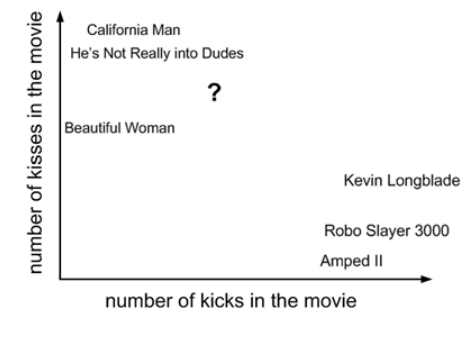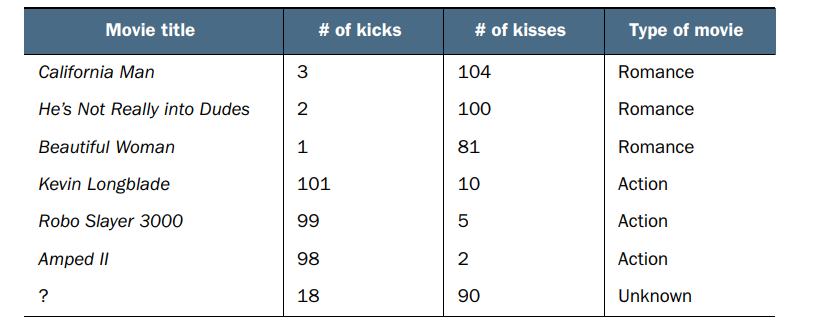# Classifying with distance measurements

k-Nearest Neighbors (kNN) works like this: we have an existing set of example data, our training set. We have labels for all of this data — we know what class each piece of the data should fall into. When we’re given a new piece of data without a label, we compare that new piece of data to the existing data, every piece of existing data. We then take the most similar pieces of data (the nearest neighbors) and look at their labels. We look at the top k most similar pieces of data from our known data-set; this is where the k comes from. (k is an integer and it’s usually less than 20.) Lastly, we take a majority vote from the k most similar pieces of data, and the majority is the new class we assign to the data we were asked to classify.

Let’s run through a quick example classifying movies into romance or action movies. Someone watched a lot of movies and counted the number of kicks and kisses in each movie. I’ve plotted six movies by the number of kisses and kicks in each movie in the following figure . Now, you find a movie you haven’t seen yet and want to know if it’s a romance movie or an action movie. To determine this, we’ll use the kNN algorithm.Classifying movies by plotting the number of kicks and kisses in each movie

We find the movie in question and see how many kicks and kisses it has. It’s plotted as a large question mark along with a few other movies in figure 2.1. These values are listed in the following tableMovies with the number of kicks and number of kisses shown for each movie, along with our assessment of the movie type

We don’t know what type of movie the question mark movie is, but we have a way of figuring that out. First, we calculate the distance to all the other movies. I’ve calculated the distances and shown those in below table. (Don’t worry about how I did these calculations right now. We’ll get into that in a few minutes.)

Now that we have all the distances to our unknown movie, we need to find the k-nearest movies by sorting the distances in decreasing order. Let’s assume k=3. Then, the three closest movies are He’s Not Really into Dudes, Beautiful Woman, and California Man. The kNN algorithm says to take the majority vote from these three movies to determine the class of the mystery movie. Because all three movies are romances, we forecast that the mystery movie is a romance movie.

# kNN in Action

First, we’ll create a Python module called kNN.py, where we’ll place all the code used in this post. The best way to learn is to start with a blank module and enter code as it’s used. First, let’s create kNN.py. We’ll create a few support functions before we create the full kNN algorithm. Add the following lines to kNN.py

In this code, we import two modules. The first one is NumPy, which is our scientific computing package. The second module is the operator module, which is used later in the kNN algorithm for sorting; we’ll get to that shortly. The function createDataSet() is there for your convenience. This creates the dataset and labels. Let’s try this out: save kNN.py, change to the directory where you’ve stored kNN.py, and launch a Python interactive session. To get started you need to open a new terminal in Linux/Mac OS or in Windows, so open a command prompt. When you’re using Linux or a Mac, you need to type python at the command line to get started, and in Windows you need to refer to the Python program directly, such as c:\Python26\python.exe, unless you have it aliased. Once you’ve started Python to load your module, you need to type

This will load the kNN module. To make sure that we’re looking at the same dataset, I created a function called createDataSet. Type the following at the Python command prompt:

This creates two variables called group and labels. To inspect each variable, type its name at the Python command prompt:

Here we have four pieces of data. Each piece of data has two attributes or features, things we know about it. In the group matrix each row is a different piece of data. Think of it as a different measurement or entry in some sort of log. As humans, we can visualize things in one, two, or sometimes three dimensions, but that’s about the limit of our brains; to keep things easy to visualize, we’ll use only two features for each data point. The label’s vector carries the labels we’ve given to each of the data points. There should be as many items in this vector as there are rows in the group matrix. We assigned the data point (1,1.1) to the class A, and similarly we assigned the data point (0,0.1) to the class B. The values in this example are arbitrarily chosen for the purpose of illustration, and the axes are unlabeled. The four data points with class labels are plotted in below figure. Now that you have an idea of how to parse and load data into Python, and you have an idea of how the kNN algorithm works, let’s put it all together and do some classification.

Pseudocode for kNN would look like this:

The Python code for the classify0() function is in the following listing.

The function classify0() takes four inputs: the input vector to classify called inX, our full matrix of training examples called dataSet, a vector of labels called labels, and, finally, k, the number of nearest neighbors to use in the voting. The labels vector should have as many elements in it as there are rows in the dataSet matrix. You calculate the distances B using the Euclidian distance where the distance between two vectors, xA and xB, with two elements, is given by

Following the distance calculation, the distances are sorted from least to greatest (this is the default). Next, C the first k or lowest k distances are used to vote on the class of inX. The input k should always be a positive integer. Lastly, D you take the classCount dictionary and decompose it into a list of tuples and then sort the tuples by the second item in the tuple using the itemgetter method from the operator module imported in the second line of the program. This sort is done in reverse so you have largest to smallest. Finally, you can return the label of the item occurring the most frequently. To predict the class, type the following text at the Python prompt:

The result should be B. Try to change the [0,0] entry to see how the answer changes. Congratulations, you just made your first classifier! You can do a lot with this simple classifier. Things will only get easier from here on out.

# Summary

The k-Nearest Neighbors algorithm is a simple and effective way to classify data. The examples in this chapter should be evidence of how powerful a classifier it is. kNN is an example of instance-based learning, where you need to have instances of data close at hand to perform the machine learning algorithm. The algorithm has to carry around the full dataset; for large datasets, this implies a large amount of storage. In addition, you need to calculate the distance measurement for every piece of data in the database, and this can be cumbersome. An additional drawback is that kNN doesn’t give you any idea of the underlying structure of the data; you have no idea what an “average” or “exemplar” instance from each class looks like. In the next posts, we’ll address this issue by exploring ways in which probability measurements can help you do classification.

--

--